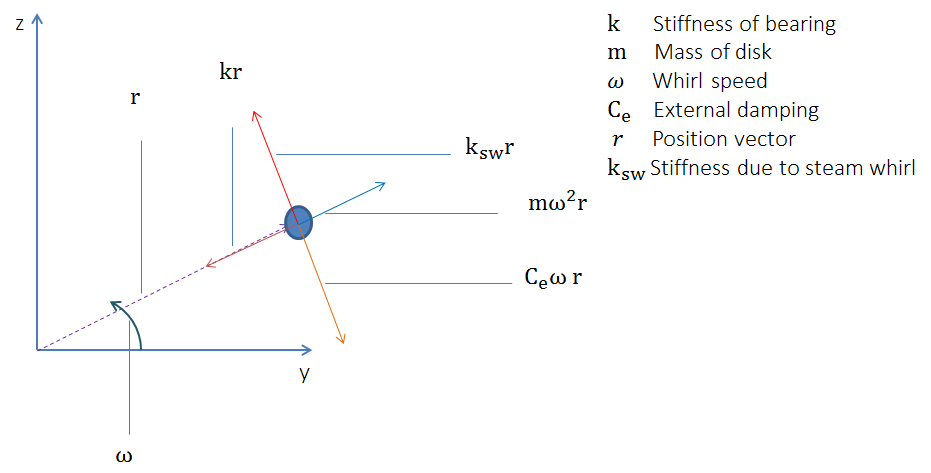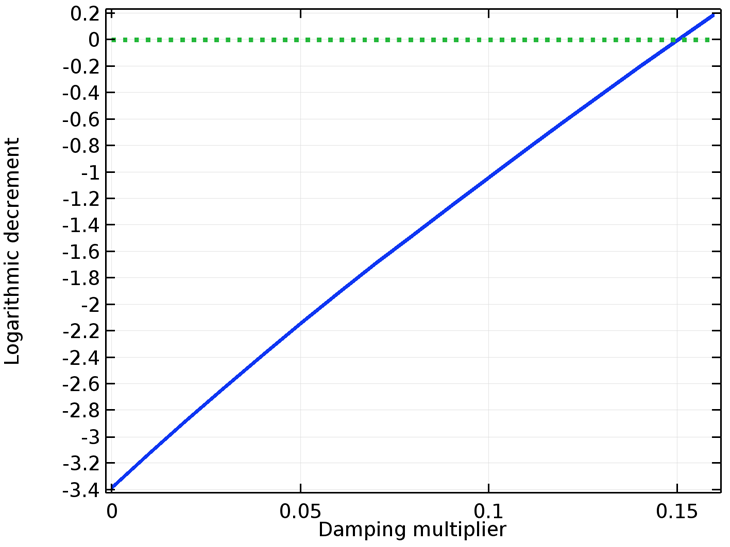# 使用 COMSOL® 软件预测转子系统的稳定性

2018年 7月 20日

### 什么是稳定性？

1. 静态
2. 动态的

• 静态稳定:扰动后，物体趋向于回到平衡位置
• 静态不稳定:物体倾向于沿着扰动的方向继续运动，而不是回到平衡位置
• 中性稳定:受扰动后，物体在扰动方向保持平衡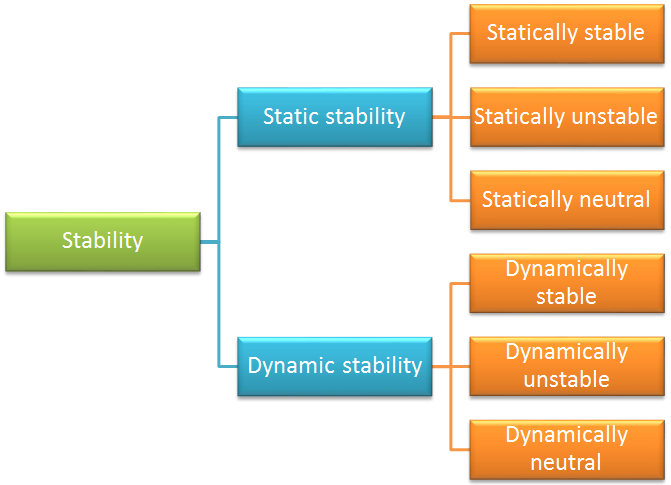• 动态稳定:系统的运动随时间而减少
• 动态不稳定:系统的运动随着时间而增加
• 动态中性:系统的运动随着时间保持不变

### 定义稳定性条件

[\textbf M]\{\ddot{\textbf u}\}+[\textbf C]\{\dot{\textbf u}\}+[\textbf K]\{\textbf u\}=\{F\}

1. 无阻尼
2. 过阻尼
3. 欠阻尼
4. 临界阻尼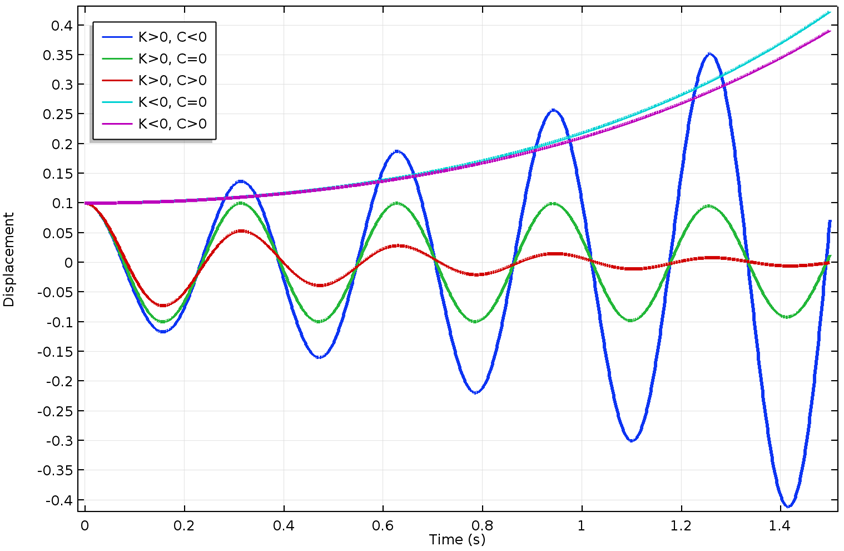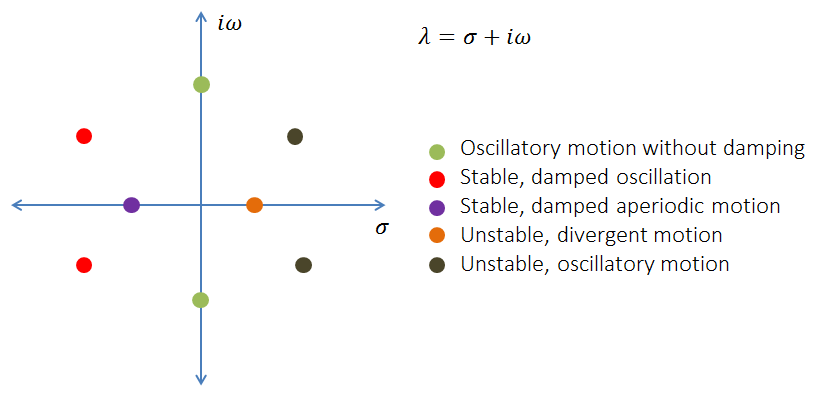\delta < 0 不稳定的
\delta > 0 稳定的

### 转子动力学问题的运动方程

#### 实心转子

[\textbf M]\{\ddot{\textbf u}\}+([\textbf C_c]+[\textbf C])\{\dot{\textbf u}\}+([\textbf K_c]+[\textbf K])\{\textbf u\}=\{F\}

#### 梁转子

[\textbf M]\{\ddot{\textbf u}\}+([\textbf C_g]+[\textbf C])\{\dot{\textbf u}\}+([\textbf K_c]+[\textbf K])\{\textbf u\}=\{F\}

### 刚度和阻尼系数如何影响转子系统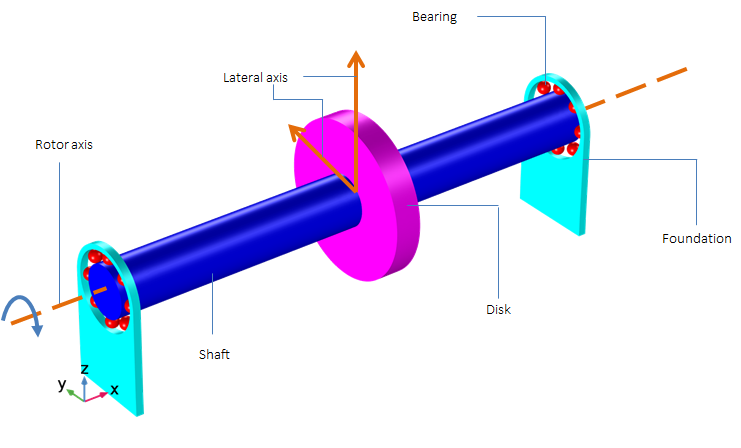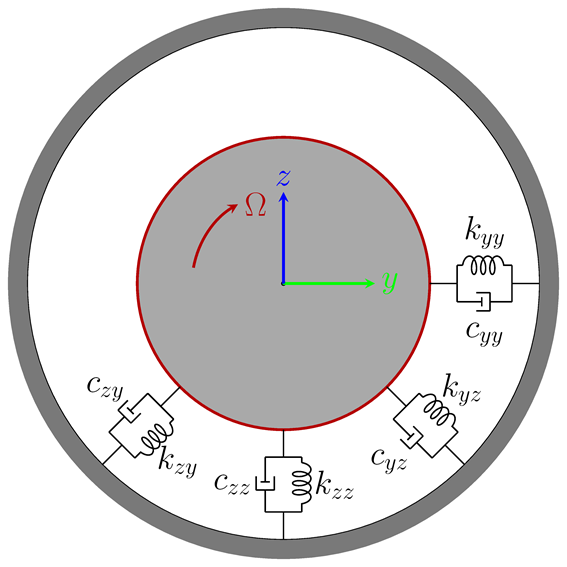\begin{Bmatrix}
\textbf F_y \\
\textbf F_z
\end{Bmatrix}=-
\begin{bmatrix}
\textbf K_{yy} & \textbf K_{yz}\\
\textbf K_{zy} & \textbf K_{zz}
\end{bmatrix}
\begin{Bmatrix}
\textbf y \\
\textbf z
\end{Bmatrix}-
\begin{bmatrix}
\textbf C_{yy} & \textbf C_{yz}\\
\textbf C_{zy} & \textbf C_{zz}
\end{bmatrix}
\begin{Bmatrix}
\mathbf{\dot{y}} \\
\mathbf{\dot{z}}
\end{Bmatrix}

\begin{matrix}
\textbf F_y = -K_{yy}\cdot\textbf y -K_{yz}\cdot\textbf z -C_{yy}\cdot \dot{\textbf y}- C_{yz}\cdot \dot{\textbf z}\\
\textbf F_z = -K_{zy}\cdot\textbf y -K_{zz}\cdot\textbf z -C_{zy}\cdot \dot{\textbf y}- C_{zz}\cdot \dot{\textbf z}
\end{matrix}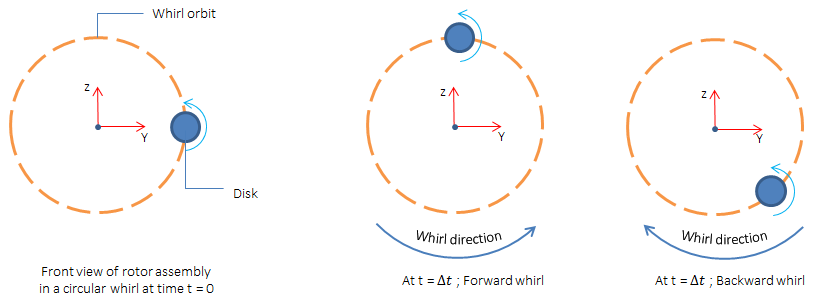#### 直接系数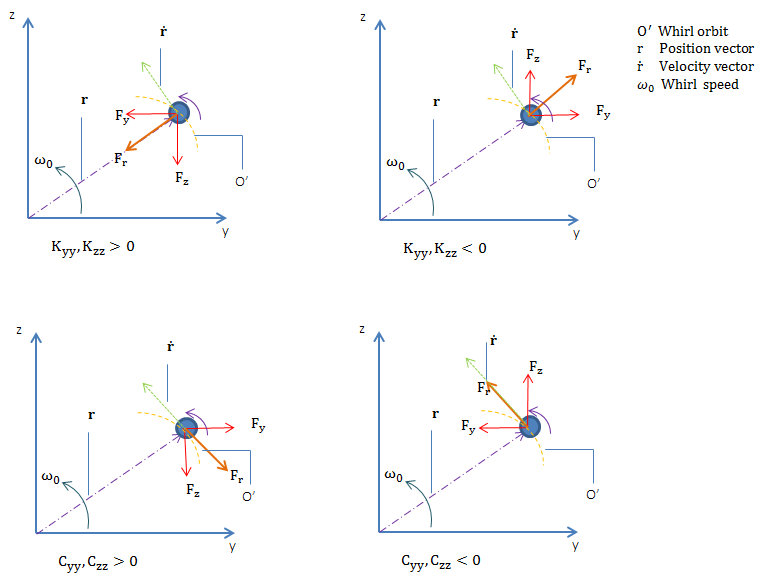#### 交叉耦合系数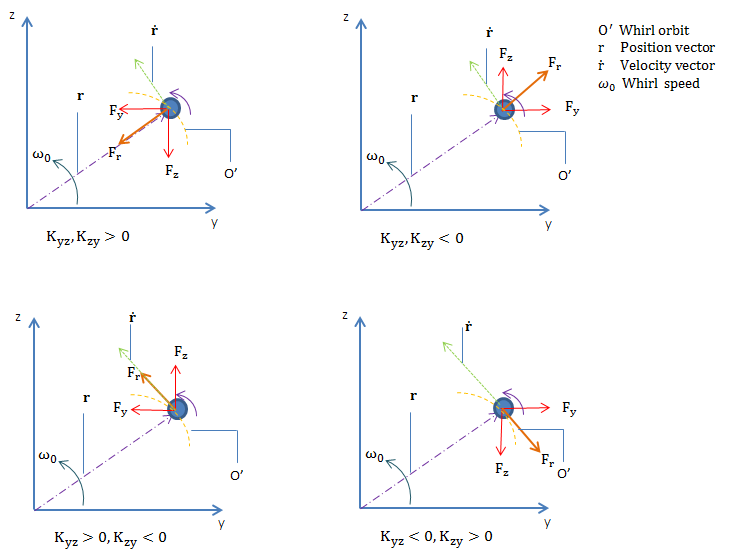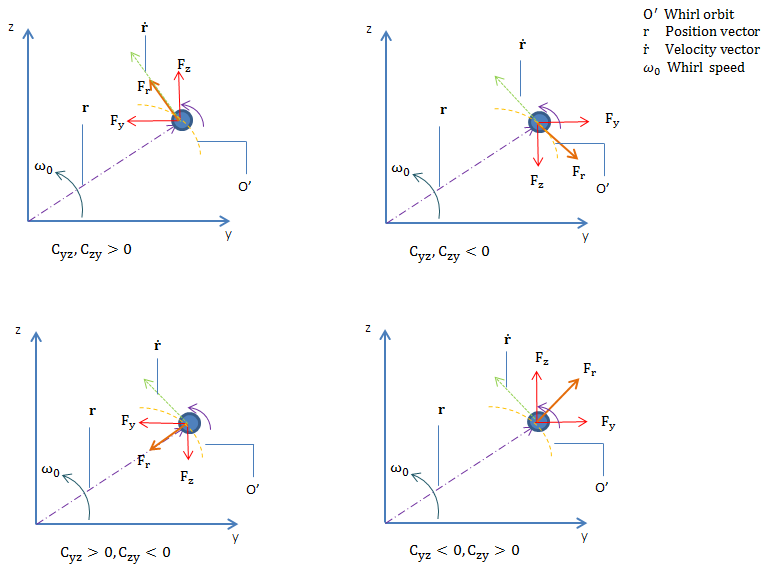\textbf K_{yy}, \textbf K_{zz} >0 稳定性处理 稳定性处理 径向
\textbf K_{yy}, \textbf K_{zz} <0 使不稳定 使不稳定 径向
\textbf K_{yz}, \textbf K_{zy} >0 稳定性处理 稳定性处理 径向
\textbf K_{yz}, \textbf K_{zy} <0 稳定性处理 稳定性处理 径向
\textbf K_{yz}>0, \textbf K_{zy}<0 使不稳定 稳定性处理 与旋转轨道相切
\textbf K_{yz}0 稳定性处理 使不稳定 与旋转轨道相切
\textbf C_{yz}, \textbf C_{zy} >0 使不稳定 使不稳定 与旋转轨道相切
\textbf C_{yz}, \textbf C_{zy} <0 稳定性处理 稳定化处理 与旋转轨道相切
\textbf C_{yy}, \textbf C_{zz} >0 稳定性处理 稳定性处理 与旋转轨道相切
\textbf C_{yy}, \textbf C_{zz} <0 使不稳定 使不稳定 与旋转轨道相切
\textbf C_{yz}>0, \textbf C_{zy}<0 稳定性处理 稳定性处理 径向
\textbf C_{yz}0 稳定性处理 稳定性处理 径向

### 转子系统稳定性分析

#### 轴承引起的不稳定性分析

\begin{Bmatrix}
\textbf F_y\\
\textbf F_z
\end{Bmatrix}=-
\begin{bmatrix}
0 &\textbf K_{yz}) \\
-\textbf K_{yz}& 0
\end{bmatrix}
\begin{Bmatrix}
\textbf y\\
\textbf z
\end{Bmatrix}

COMSOL案例库中的模型讨论了交叉耦合力引起的稳定性。该模型包括一个涡轮增压器转子，由两个轴承支撑:一个靠近压缩机，另一个靠近涡轮机，使压缩机和涡轮机都悬挂在轴上。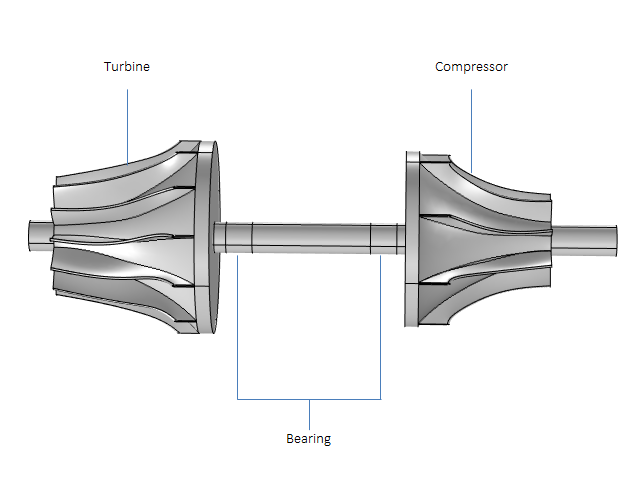1. 如果交叉耦合刚度已知，它可以直接被指定为 轴承节点下的非对角项
2. 如果力是已知的，可以在轴承 节点 使用 力和力矩 选项
3. 为了捕捉轴承的完全非线性效应，可以直接将转子模拟与流体动力轴承模拟耦合起来

#### 旋转阻尼引起的不稳定性

\begin{Bmatrix}
\mathbf{F_y}\\
\mathbf{F_z}
\end{Bmatrix}=
\begin{bmatrix}
\mathbf{C_i}&\mathbf{0} \\
\mathbf{0}& \mathbf{C_i}
\end{bmatrix}
\begin{Bmatrix}
\mathbf{\dot{y}}\\
\mathbf{\dot{z}}
\end{Bmatrix}
+
\begin{bmatrix}
\mathbf{0}&\mathbf{(C_i\cdot\omega)} \\
\mathbf{-(C_i\cdot\omega)}& 0
\end{bmatrix}
\begin{Bmatrix}
\mathbf{y}\\
\mathbf{z}
\end{Bmatrix}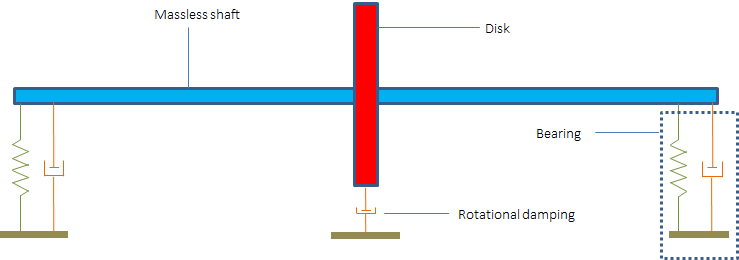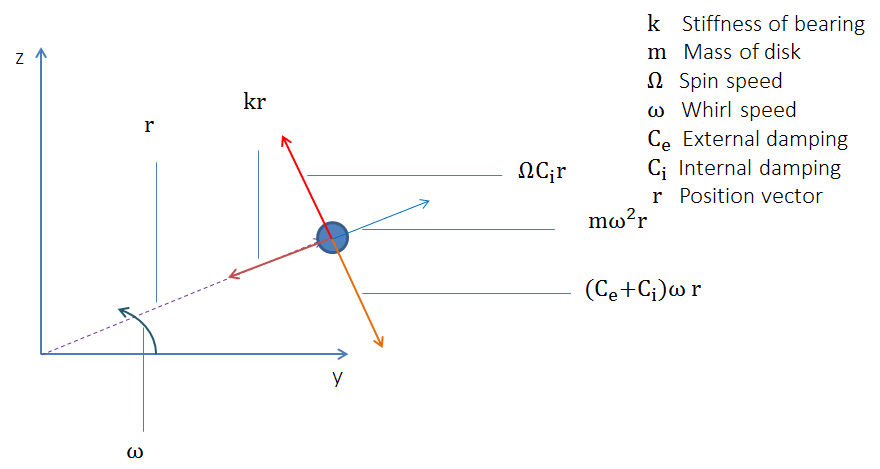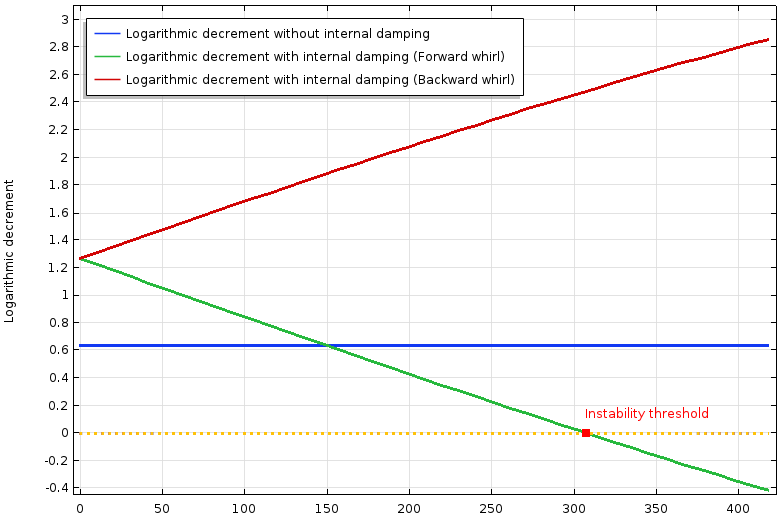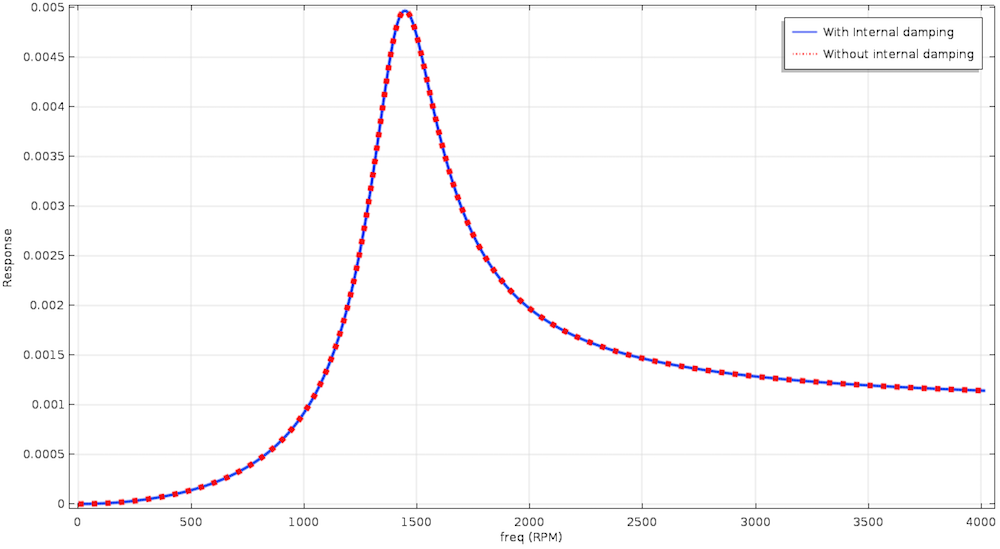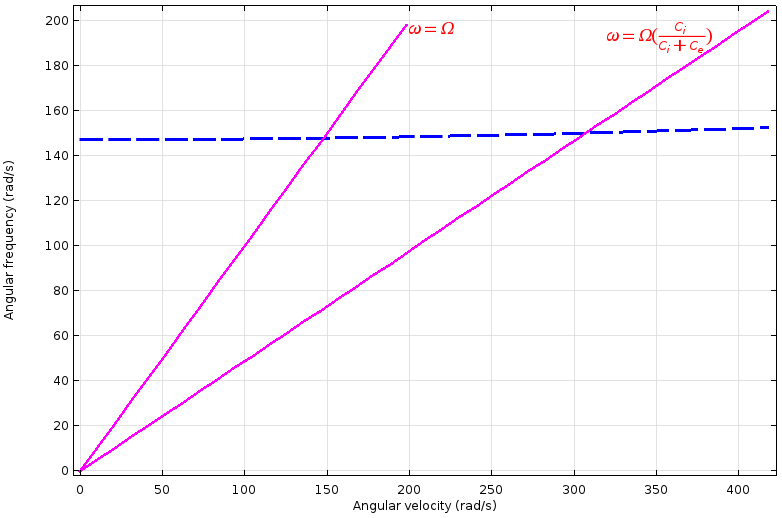#### 蒸汽涡动引起的不稳定性

\begin{Bmatrix}
\mathbf{F_y}\\
\mathbf{F_z}
\end{Bmatrix}=
\begin{bmatrix}
\mathbf{0}&\mathbf{(K_{sw})} \\
-\mathbf{(K_{sw})}& 0
\end{bmatrix}
\begin{Bmatrix}
\mathbf{y}\\
\mathbf{z}
\end{Bmatrix}